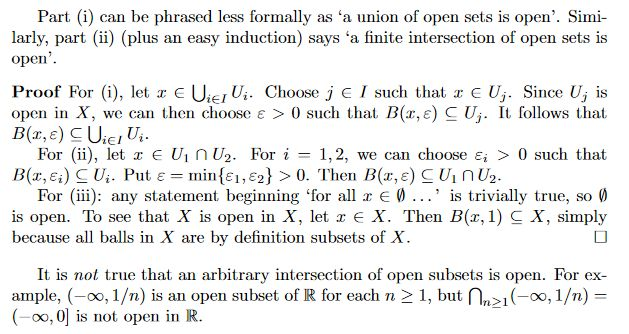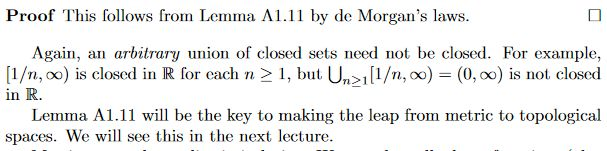Show Question
Math and science::Topology

# Metric space. Unions and intersections of open sets

• An arbitrary union of open subsets is open.
• A finite intersection of open subsets is open.
• Special cases: $$\emptyset$$ and $$X$$ are both open and closed.

### Lemma. Union and intersection of open sets

Let $$X$$ be a metric space.

• Let $$(U_i)_{i \in I}$$ be a family (finite or not) of open subsets of $$X$$. Then $$\bigcup_{i\in I} U_i$$ is also open in X.
• Let $$U_1$$ and $$U_2$$ be open subsets of $$X$$. Then $$U_1 \cap U_2$$ is also open in X.
• Special cases $$\emptyset$$ and $$X$$ are open also.The mirror statements for closed sets are as follows.

### Lemma. Union and intersection of closed subsets

Let $$X$$ be a metric space.

• Let $$V_1$$ and $$V_2$$ be closed subsets of $$X$$. Then $$V_1 \cup V_2$$ is also closed in X.
• Let $$(V_i)_{i \in I}$$ be a family (finite or not) of closed subsets of $$X$$. Then $$\bigcap_{i\in I} V_i$$ is also open in X.

Special cases $$\emptyset$$ and $$X$$ are also closed.## Context#### Source

Tom Leinster's notes